# CBSE Physics for Class 12 | Electrostatics | Electrical Field

## Electrical Field

A positive charge or a negative charge is said to create its field around itself.

If a charge$\displaystyle {{Q}_{1}}$ exerts a force on charge$\displaystyle {{Q}_{2}}$placed near it, it may be stated that since$\displaystyle {{Q}_{2}}$ is in the field of$\displaystyle {{Q}_{1}}$, it experiences some force, or it may also be said that since charge$\displaystyle {{Q}_{1}}$is inside the field of$\displaystyle {{Q}_{2}}$, it experience some force.

Thus space around a charge in which another charged particle experiences a force is said to have electrical field in it.

### (1) Electric field intensity$\displaystyle (\vec{E})$:

The electric field intensity at any point is defined as the force experienced by a unit positive charge placed at that point.$\displaystyle \vec{E}= \frac{{\vec{F}}}{{{q}_{0}}}$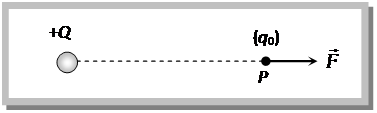Where$\displaystyle {{q}_{0}}\to 0$so that presence of this charge may not affect the source charge Q and its electric field is not changed, therefore expression for electric field intensity can be better written as$\displaystyle \vec{E}=\underset{{{q}_{0}}\to 0}{\mathop{Lim}}\,\,\,\,\frac{{\vec{F}}}{{{q}_{0}}}$

### (2) Unit and Dimensional formula of Electrical Field :

It’s S.I. unit –$\displaystyle \frac{Newton}{coulomb}=\frac{volt}{meter}=\frac{Joule}{coulomb\times meter}$ and

C.G.S. unit – Dyne/stat coulomb.

Dimension :
[$\displaystyle E$] =[$\displaystyle ML{{T}^{-3}}{{A}^{-1}}$]

### (3) Direction of electric field :

Electric field (intensity)$\displaystyle \vec{E}$ is a vector quantity. Electric field due to a positive charge is always away from the charge and that due to a negative charge is always towards the charge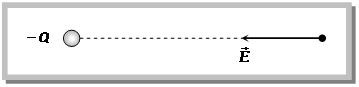### (4) Relation between electric force and electric field :

In an electric field$\displaystyle \vec{E}$ a charge (Q) experiences a force$\displaystyle F=QE$. If charge is positive then force is directed in the direction of field while if charge is negative force acts on it in the opposite direction of field### (5) Superposition of electric field (electric field at a point due to various charges) :

The resultant electric field at any point is equal to the vector sum of electric fields at that point due to various charges.$\displaystyle \vec{E}={{\vec{E}}_{1}}+{{\vec{E}}_{2}}+{{\vec{E}}_{3}}+...$

The magnitude of the resultant of two electric fields is given by$\displaystyle E=\sqrt{E_{1}^{2}+E_{2}^{2}+2{{E}_{1}}{{E}_{2}}\cos \theta }$ and the direction is given by$\displaystyle \tan \alpha =\frac{{{E}_{2}}\sin \theta }{{{E}_{1}}+{{E}_{2}}\cos \theta }$

### (6) Electric field due to continuous distribution of charge :

A system of closely spaced electric charges forms a continuous charge distribution

#### Linear charge distribution

In this distribution charge distributed on a line.

For example : charge on a wire, charge on a ring etc. Relevant parameter is$\displaystyle \lambda$ which is called linear charge density i.e.,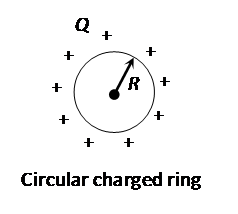$\displaystyle \lambda =\frac{\text{charge}}{\text{length}}$$\displaystyle \lambda =\frac{Q}{2\pi R}$

#### Surface charge distribution

In this distribution charge distributed on the surface.

For example : Charge on a conducting sphere, charge on a sheet etc. Relevant parameter is$\displaystyle \sigma$which is called surface charge density i.e.,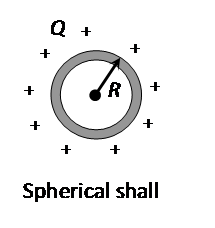$\displaystyle \sigma =\frac{\text{charge}}{\text{area}}$$\displaystyle \sigma =\frac{Q}{4\pi {{R}^{2}}}$

#### Volume charge distribution

In this distribution charge distributed in the whole volume of the body.

For example : Non conducting charged sphere. Relevant parameter is$\displaystyle \rho$ which is called volume charge density i.e.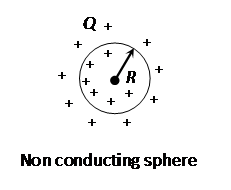$\displaystyle \rho =\frac{\text{charge}}{\text{volume}}$$\displaystyle \rho =\frac{Q}{\frac{4}{3}\pi {{R}^{3}}}$

To find the field of a continuous charge distribution, we divide the charge into infinitesimal charge elements.

Each infinitesimal charge element is then considered, as a point charge and electric field$\displaystyle \overrightarrow{dE}$ is determined due to this charge at given point.

The Net field at the given point is the summation of fields of all the elements. i.e.,$\displaystyle \overrightarrow{E\,}=\int{\overrightarrow{dE}}$

# CBSE Physics for Class 12 | Electrostatics | Coulomb’s Law

## Coulomb’s Law.

If two stationary and point charges$\displaystyle Q{}_{1}$ and$\displaystyle Q{}_{2}$ are kept at a distance r, then it is found that force of attractionor repulsion between them is$\displaystyle F\propto \frac{Q{}_{1}Q{}_{{{2}_{{}}}}}{{{r}^{2}}}$ i.e.,$\displaystyle F=\frac{kQ{}_{1}Q{}_{2}}{{{r}^{2}}}$ ; (k = Proportionality constant)

### (1) Dependence of k :

Constant k depends upon system of units and medium between the two charges.

#### (i) Effect of units

(a) In C.G.S. for air$\displaystyle k=1,$$\displaystyle F=\frac{{{Q}_{1}}\,{{Q}_{2}}}{{{r}^{2}}}$ Dyne

(b) In S.I. for air$\displaystyle k=\frac{1}{4\pi {{\varepsilon }_{0}}}=9\times {{10}^{9}}\frac{N-m{}^{2}}{C{}^{2}}$,$\displaystyle F=\frac{1}{4\pi {{\varepsilon }_{0}}}.\frac{{{Q}_{1}}{{Q}_{2}}}{{{r}^{2}}}$Newton (1 Newton = 105
Dyne)

Note :

•$\displaystyle {{\varepsilon }_{0}}=$Absolute permittivity of air or free space =$\displaystyle 8.85\times {{10}^{-12}}\frac{{{C}^{2}}}{N-{{m}^{2}}}$$\displaystyle \left( =\frac{Farad}{m} \right)$. It’s Dimension is$\displaystyle [M{{L}^{-3}}{{T}^{4}}{{A}^{2}}]$
•$\displaystyle {{\varepsilon }_{0}}$Relates with absolute magnetic permeability ($\displaystyle {{\mu }_{0}}$) and velocity of light (c) according to the following relation$\displaystyle c=\frac{1}{\sqrt{{{\mu }_{0}}{{\varepsilon }_{0}}}}$

#### (ii) Effect of medium

(a) When a dielectric medium is completely filled in between charges rearrangement of the charges inside the dielectric medium takes place and the force between the same two charges decreases by a factor of K known as dielectric constant or specific inductive capacity (SIC) of the medium, K is also called relative permittivity er of the medium (relative means with respect to free space).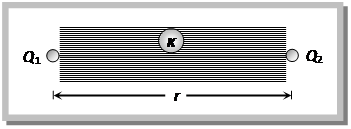Hence in the presence of medium$\displaystyle {{F}_{m}}=\frac{{{F}_{air}}}{K}=\frac{1}{4\pi {{\varepsilon }_{0}}K}.\,\frac{{{Q}_{1}}{{Q}_{2}}}{{{r}^{2}}}$

Here$\displaystyle {{\varepsilon }_{0}}K={{\varepsilon }_{0}}\,{{\varepsilon }_{r}}=\varepsilon$ (permittivity of medium)

(b) If a dielectric medium (dielectric constant K, thickness t) is partially filled between the charges then effective air separation between the charges becomes$\displaystyle (r-t\,+t\sqrt{K})$Hence force$\displaystyle F=\frac{1}{4\pi {{\varepsilon }_{0}}}\,\frac{{{Q}_{1}}{{Q}_{2}}}{{{(r-t+t\sqrt{K})}^{2}}}$

### (2) Vector form of coulomb’s law :

Vector form of Coulomb’s law is$\displaystyle {{\overrightarrow{F\,}}_{12}}=K.\frac{{{q}_{1}}{{q}_{2}}}{{{r}^{3}}}{{\overrightarrow{\,r}}_{12}}=K.\frac{{{q}_{1}}{{q}_{2}}}{{{r}^{2}}}{{\hat{r}}_{12}},$

where$\displaystyle {{\hat{r}}_{12}}$ is the unit vector from first charge to second charge along the line joining the two charges.

### (3) A comparative study of fundamental forces of nature

 S.No. Force Nature and formula Range Relative strength (i) Force of gravitation between two masses Attractive F = Gm1m2/r2, obey’s Newton’s third law of motion, it’s a conservative force Long range (between planets and between electron and proton) 1 (ii) Electromagnetic force (for stationary and moving charges) Attractive as well as repulsive, obey’s Newton’s third law of motion, it’s a conservative force Long (upto few kelometers)$\displaystyle {{10}^{37}}$ (iii) Nuclear force (between nucleons) Exact expression is not known till date. However in some cases empirical formula$\displaystyle {{U}_{0}}{{e}^{r/{{r}_{0}}}}$ can be utilized for nuclear potential energy$\displaystyle {{U}_{0}}$ and$\displaystyle {{r}_{0}}$ are constant. Short (of the order of nuclear size 10–15 m) 1039 (strongest) (iv) Weak force (for processes like b decay) Formula not known Short (upto 10–15m) 1024

Note :

• Coulombs law is not valid for moving charges because moving charges produces magnetic field also.
• Coulombs law is valid at a distance greater than$\displaystyle {{10}^{-15}}m.$
• A charge$\displaystyle {{Q}_{1}}$exert some force on a second charge$\displaystyle {{Q}_{2}}$. If third charge$\displaystyle {{Q}_{3}}$ is brought near, the force of$\displaystyle {{Q}_{1}}$ exerted on$\displaystyle {{Q}_{2}}$ remains unchanged.
• Ratio of gravitational force and electrostatic force between (i) Two electrons is 10–43/1. (ii) Two protons is 10–36/1 (iii) One proton and one electron 10–39/1.
• Decreasing order to fundamental forces$\displaystyle {{F}_{Nuclear}}>{{F}_{Electromagnetic}}>{{F}_{Weak}}>{{F}_{Gravitational}}$

### (4) Principle of superposition :

According to the principle of super position, total force acting on a given charge due to number of charges is the vector sum of the individual forces acting on that charge due to all the charges.Consider number of charge$\displaystyle {{Q}_{1}}$,$\displaystyle {{Q}_{2}}$,$\displaystyle {{Q}_{3}}$…are applying force on a charge Q

Net force on Q will be$\displaystyle {{\vec{F}}_{net}}={{\vec{F}}_{1}}+{{\vec{F}}_{2}}+..........+{{\vec{F}}_{n-1}}+{{\vec{F}}_{n}}$